# How to Count Dates of Given Year in Excel

This post will guide you how to count Dates of a certain year in the range of dates using a formula in Excel 2013/2016 or Excel office 365. How do I count dates by a given year in Excel. And you may be want to count the cells where dates are from a particular year. It should be similar with the previous post that how to count dates by day of week. And we can use the SUMPRODUCT function and the YEAR function to build a formula to count the date values where the date belongs the specific given year.

## Count Dates of Given Year Through Formula

Assuming that you may be want to count dates of given year in date range B1:B6. You can use the below formula based on the SUMPRODUCT function and the YEAR function, like this:

=SUMPRODUCT(–(YEAR(\$B\$1:\$B\$6)=D2))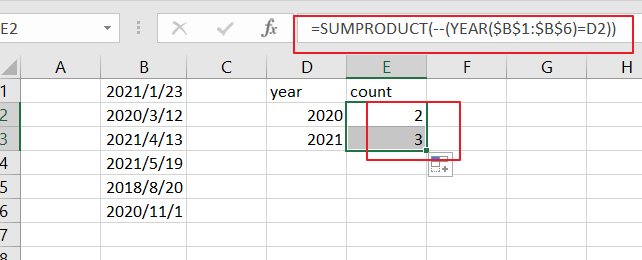Note: you need to change date range as you need. And changing year value given as cell reference.

LETS SEE THAT HOW THIS FORMULA WORKS

=YEAR(B1：B6)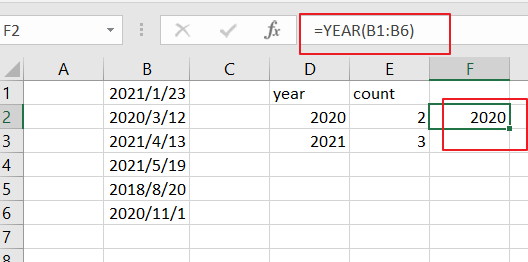The YEAR function will extract the year value from the given data range. And it returns an array result like this:

{2021;2020;2021;2021;2018;2020}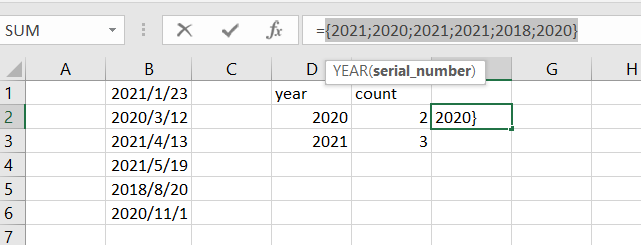=YEAR(B1：B6)=D2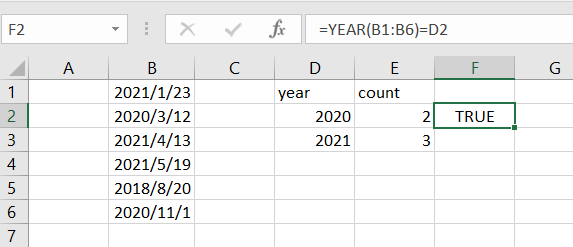Then the array results returned by YEAR formula are compared to the given year value, and returns another array result of TRUE and FALSE values like this:

{FALSE;TRUE;FALSE;FALSE;FALSE;TRUE}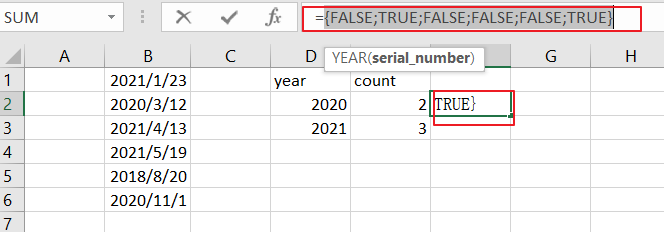=–(YEAR(B1:B6)=D2)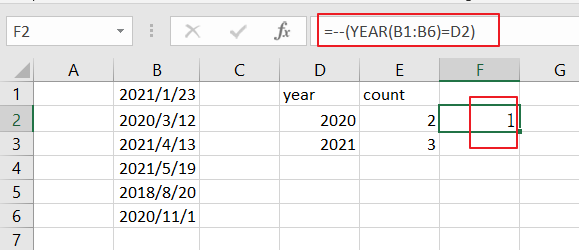You still need to convert the TRUE or FALSE values to 1 and 0 values using the double negative operator. And returns the below array result:

{1;0;0;0;0;0}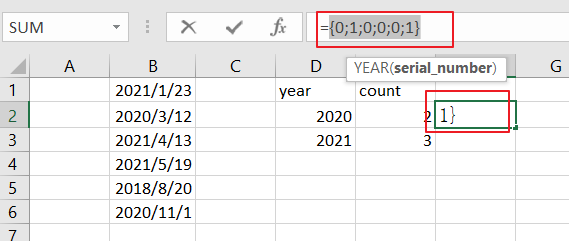The SUMPRODUCT function just sums all values of an array returned by the above formula.

You can see that the total date matches of the year 2020 comes out to be 2. And it means that there are 2 date values where the year value equals to 2020.

### Related Functions

• Excel SUMPRODUCT function
The Excel SUMPRODUCT function multiplies corresponding components in the given one or more arrays or ranges, and returns the sum of those products. The syntax of the SUMPRODUCT function is as below:= SUMPRODUCT (array1,[array2],…)…
• Excel YEAR function
The Excel YEAR function returns a four-digit year from a given date value, the year is returned as an integer ranging from 1900 to 9999. The syntax of the YEAR function is as below:=YEAR (serial_number)…